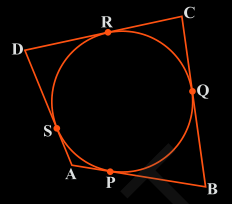# A quadrilateral ABCD is drawn to circumscribe a circle (see Fig.) . Prove that AB + CD = AD + BCLet the P, Q, R and S be point of contacts for tangent AB, BC, CD and DA respectively .

AP = AS ……..(1)

BP = BQ ……..(2)

CR = CQ ……..(3)

DR = DS ……..(4)

By, adding (1), (2), (3) and (4) RHS = LHS, we get

AP + BP + CR + DR = AS + BQ + CQ + DS

(AP + BP) + (CR + DR) = (AS + DS) + (BQ + CQ) (On Rearranging)

AB + CD = AD + BC

Hence, proved !!